## 12.1.1 The Information Space as a Big State Space

Recall that any problem specified using Formulation 11.1 can be converted using derived I-states into a problem under Formulation 10.1. By building on the discussion from the end of Section 11.1.3, this can be achieved by treating the I-space as a big state space in which each state is an I-state in the original problem formulation. Some of the components were given previously, but here a complete formulation is given.

Suppose that a problem has been specified using Formulation 11.1, resulting in the usual components:,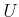,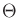,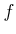,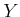,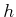,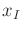,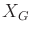, and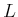. The following concepts will work for any sufficient I-map; however, the presentation will be limited to two important cases: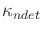and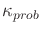, which yield derived I-spaces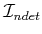and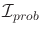, respectively (recall Sections 11.2.2 and 11.2.3).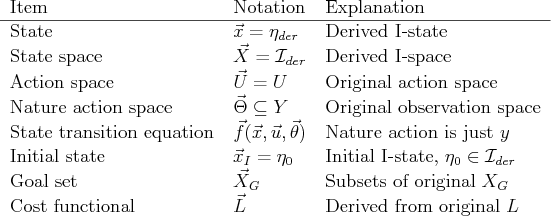The components of Formulation 10.1 will now be specified using components of the original problem. To avoid confusion between the two formulations, an arrow will be placed above all components of the new formulation. Figure 12.1 summarizes the coming definitions. The new state space,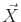, is defined as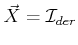, and a state,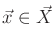, is a derived I-state,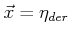. Under nondeterministic uncertainty,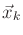means, in which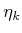is the history I-state. Under probabilistic uncertainty,means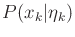. The action space remains the same: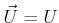.

The strangest part of the formulation is the new nature action space,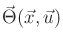. The observations in Formulation 11.1 behave very much like nature actions because they are not selected by the robot, and, as will be seen shortly, they are the only unpredictable part of the new state transition equation. Therefore,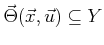, the original observation space. A new nature action,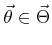, is just an observation,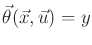. The setgenerally depends on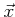andbecause some observations may be impossible to receive from some states. For example, if a sensor that measures a mobile robot position is never wrong by more thanmeter, then observations that are further thanmeter from the true robot position are impossible.

A derived state transition equation is defined with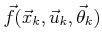and yields a new state,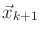. Using the original notation, this is just a function that uses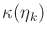,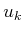, and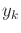to compute the next derived I-state,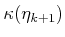, which is allowed because we are working with sufficient I-maps, as described in Section 11.2.1.

Initial states and goal sets are optional and can be easily formulated in the new representation. The initial I-state,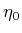, becomes the new initial state,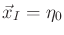. It is assumed thatis either a subset ofor a probability distribution, depending on whether planning occurs inor. In the nondeterministic case, the new goal set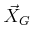can be derived as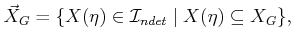(12.1)

which is the set of derived I-states for which it is guaranteed that the true state lies in. A probabilistic version can be made by requiring that all states assigned nonzero probability by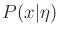lie in. Instead of being nonzero, a threshold could be used. For example, the goal may require being only 98% certain that the goal is reached.

The only remaining portion of Formulation 10.1 is the cost functional. We will develop a cost model that uses only the state and action histories. A dependency on nature would imply that the costs depend directly on the observation,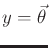, which was not assumed in Formulation 11.1. The general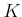-stage cost functional from Formulation 10.1 appears in this context as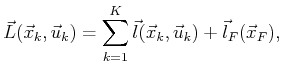(12.2)

with the usual cost assumptions regarding the termination action.

The cost functional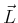must be derived from the cost functionalof the original problem. This is expressed in terms of states, which are unknown. First consider the case of. The state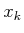at stagefollows the probability distribution, as derived in Section 11.2.3. Using, an expected cost is assigned as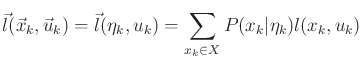(12.3)

and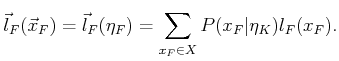(12.4)

Ideally, we would like to make analogous expressions for the case of; however, there is one problem. Formulating the worst-case cost for each stage is too pessimistic. For example, it may be possible to obtain high costs in two consecutive stages, but each of these may correspond to following different paths in. There is nothing to constrain the worst-case analysis to the same path. In the probabilistic case there is no problem because probabilities can be assigned to paths. For the nondeterministic case, a cost functional can be defined, but the stage-additive property needed for dynamic programming is destroyed in general. Under some restrictions on allowable costs, the stage-additive property is preserved.

The stateat stageis known to lie in, as derived in Section 11.2.2. For every history I-state,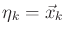, and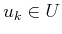, assume that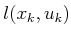is invariant over all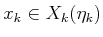. In this case,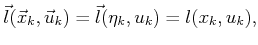(12.5)

in which, and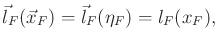(12.6)

in which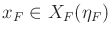.

A plan on the derived I-space,or, can now also be considered as a plan on the new state space. Thus, state feedback is now possible, but in a larger state spaceinstead of. The outcomes of actions are still generally unpredictable due to the observations. An interesting special case occurs when there are no observations. In this case, the I-state is predictable because it is derived only from actions that are chosen by the robot. In this case, the new formulation does not need nature actions, which reduces it down to Formulation 2.3. Due to this, feedback is no longer needed if the initial I-state is given. A plan can be expressed once again as a sequence of actions. Even though the original states are not predictable, the future information states are! This means that the state trajectory in the new state space is completely predictable as well.

Steven M LaValle 2020-08-14